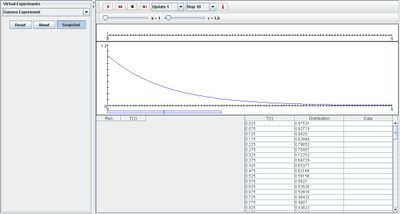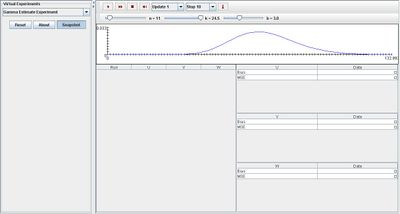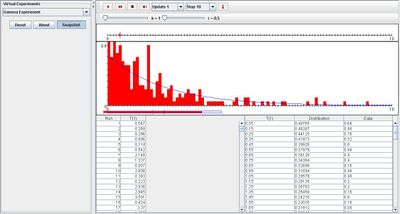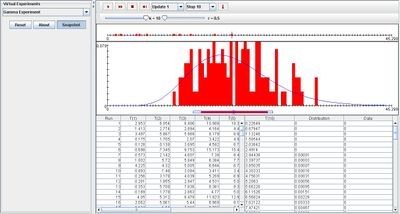# SOCR EduMaterials Activities GammaExperiment

## Description

The Gamma Experiment consists of running a Poisson process until the k’th arrival. The arrivals are shown as red dots in the timeline. The density function of the k’th arrival Tk is shown in the graph box, and the moments are given in the distribution table. The arrival times are recorded in the update table. The parameters are the rate of the process r and the arrival number k, which can be varied with scroll bars.

## Goal

To provide a simulation for Gamma Distribution that is analogous to the Poisson Process, and allows others to gain a better understanding of the Gamma Distribution.

## Experiment

Go to the SOCR Experiment [] and select the Gamma Experiment from the drop-down list of experiments on the top left. The image below shows the initial view of this experiment:When pressing the play button, one trial will be executed and recorded in the distribution table below. The fast forward button symbolizes the nth number of trials to be executed each time. The stop button ceases any activity and is helpful when the experimenter chooses “continuous,” indicating an infinite number of events. The fourth button will reset the entire experiment, deleting all previous information and data collected. The “update” scroll indicates nth number of trials (1, 10, 100, or 1000) performed when selecting the fast forward button and the “stop” scroll indicates the maximum number of trials in the experiment.

When varying k, the distribution graph in blue changes shape. Initially, it is skewed right, but once k increases, a peak begins to form and moves toward the right of the graph as it continues to increase. The maximum of k shows a peak in the middle of the distribution graph. Varying parameter r changes the values of the x and y axes. The image below displays this characteristic:Note that when k has a small value, the empirical density graph shown in red has a large spread. When k has a large value, the empirical density graph has a much smaller spread that is saturated at the peak of the distribution graph in blue. The two images shown below demonstrate this effect:## Applications

The Gamma Experiment is a general applet that demonstrates the basic properties of the Gamma Distribution. It is in a similar form to the Poisson process in which the probabilities of events are usually based on the time of the first success and the number of successes per time period. This simulation may be used in many different kinds of events such as:

Suppose scientists want to determine the probability of an earthquake to occur within the next two years. Given the number of earthquakes in the past 100 years, they determine the number of successes per year (time period). By using this applet, the scientists have the ability to analytically and graphically display their findings to others.

For instance, a machine measures the weight of boxes. Workers of the factory want to determine the probability of a box exceeding the interval of weight for the boxes. They are given information regarding the number of successes (boxes exceeding the weight limit) and are able to calculate their probability of interest. Using this applet will give them the advantage to generalize their findings in a simple and easy manner.